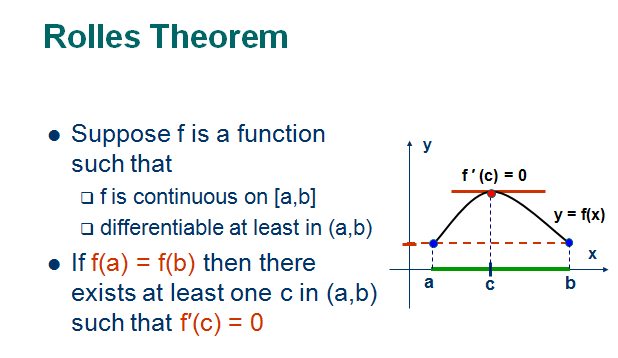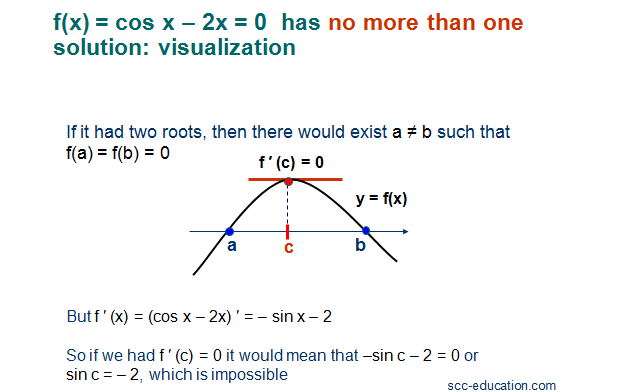# SCC Education

## Rolle’s theorem and Mean Value Theorem

Rolle’s theorem and Mean Value Theoremsome-important-formulas-of-integration

solving-models-related-to-ordinary differential equation

differentiation-of-exponential-functions

area-between-curve

vector

application-of-derivatives

homogenous-differential-equation

inverse-trigonometry-questions

integration

integration-questions

Rolles-theorem-and-mean-value-theorem

Techniques-of-integration-substitution

critical-points-and-point-of-inflection

intermediate-value-theorem

#### 1 comment:

1.You have given valuable data for us. It is excellent and informative for everyone. Keep posting always. I am very thankful to you. buy soundcloud followers